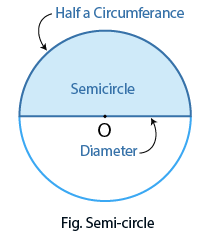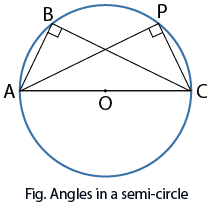Angle in a semicircle

## Objective

To verify that angle in a semi-circle is a right angle, using vector method.

## Semi-circle

A semi-circle is half a circle. The curved edge is half the circumference, and the straight edge is the diameter, i.e., refer to Fig. (Semi-circle).## What are the angles in a semi-circle?• Angles in a semi-circle are created when you join the two ends of the diameter to one point on the arc using chords.
• In the Fig. (Angles in a semi-circle), AC is the diameter of a circle that divides the circle into two.
• The two points A and C are joined to points B and P on the circumference using two chords.
• This triangle is a right-angle triangle with a 90° angle touching the arc. This is the same for any point that is placed on the arc.

## Definition of the dot product

• The dot product, also known as the scalar product or inner product, is an operation that takes two vectors and produces a scalar (a real number).
• The dot product of two vectors, denoted as A ⋅ B, is given by the formula:
• A ⋅ B = |A| |B| cos(θ)
• Where |A| and |B| represent the magnitudes (or lengths) of vectors A and B, respectively, and θ is the angle between the vectors.

## Perpendicular vectors and the dot product

• If two vectors A and B are perpendicular to each other (i.e., they form a right angle), the dot product between them is zero.
• This can be seen from the dot product formula:
• A ⋅ B = |A| |B| cos(90°) = |A| |B| (0) = 0

## Vector representation in a semi-circle

• When working with angles in a semi-circle using the vector method, we typically consider the radius vector and the tangent vector at the point of contact.
• The radius vector is a line segment from the centre of the circle to the point on the semi-circle, while the tangent vector is perpendicular to the radius at the point of contact.